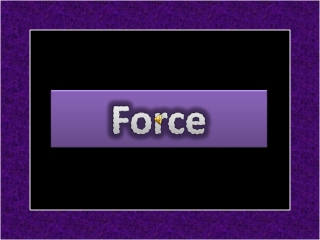DownloadDownload PresentationForce

Force

Download PresentationForce

- - - - - - - - - - - - - - - - - - - - - - - - - - - E N D - - - - - - - - - - - - - - - - - - - - - - - - - - -
Presentation Transcript

1. Force

2. What is Force? • It is measured in the SI unit of newtons and represented by the symbol F. • a force is any influence that causes an objectto undergo a certain change, either concerning its movement, direction, or geometrical construction. • Formula: F=ma

3. Pre-Newtonian Concepts • Aristotle famously described a force as anything that causes an object to undergo "unnatural motion. • Aristotle provided a philosophical discussion of the concept of a force as an integral part of Aristotelian cosmology.

4. Laws of Motion • First Law of Motion • Newton's First Law of Motion states that objects continue to move in a state of constant velocity unless acted upon by an external net force or resultant force. • Second Law of Motion • A modern statement of Newton's Second Law is a vector differential equation. • Third Law of Motion • For every action there is an equal and opposite reaction.

5. First Law of Motion

6. Second Law of Motion

7. Third Law of Motion

8. Pre-Newtonian Concepts • Galileo Galileiwas the first to point out the inherent contradictions contained in Aristotle's description of forces. • First described the Dynamic equilibrium.

9. What is Gravity? • It is introduced by Isaac Newton. • Formula: F=mg • The force of gravity is opposed by the reactions of their supports.

10. Static equilibrium was understood well before the invention of classical mechanics. The simplest case of static equilibrium occurs when two forces are equal in magnitude but opposite in direction. Equilibrium occurs when the resultant force acting on a point particle is zero (that is, the vector sum of all forces is zero).

11. The Electrostatic force was first described in 1784 by Coulomb as a force which existed intrinsically between two charges.